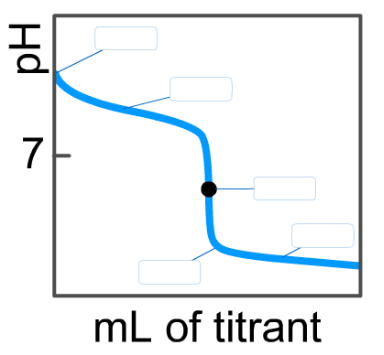# Problem: On the weak base/strong acid titration curve below, label the following points.a) The point where the pH corresponds to a solution of the weak base (B) in water.b) The point where the pH corresponds to a solution of the conjugate acid (BH +) in water.c) The point where pH=pKa (for BH+).

###### FREE Expert Solution
97% (194 ratings)
###### FREE Expert Solution

We are asked to look for the parts described in the diagram given.

Let analyze each statement one by one.

(a) The point where the pH corresponds to a solution of the weak base (B) in water.

● pH is determined only by the concentration of B → no titrant (strong acid) is added to the solution yet

corresponds to the starting point

97% (194 ratings)###### Problem Details

On the weak base/strong acid titration curve below, label the following points.

a) The point where the pH corresponds to a solution of the weak base (B) in water.

b) The point where the pH corresponds to a solution of the conjugate acid (BH +) in water.

c) The point where pH=pK(for BH+).